# Ray Optics

The form of energy that enables us to see the objects around us is called light. The branch of Physics that deals with the nature, properties, sources and the effects of light is called optics. Optics is broadly divided into two branches, namely physical optics, which is the study of the wave-like nature of light and the interactions between light and matter. Ray optics, also known as geometrical optics, is the study of simple properties of light and optical instruments by assuming that light travels in a straight line.

Ray optics deals with the geometry of the light. Light always travels in a straight line and the direction in which the light rays propagate is called the ray of light. In ray optics, we will study the image formed by the mirrors, lens and prisms.

## Important terms related to mirror

1. Pole: The centre point of the mirror through which the principal axis passes through the mirror is called the pole.

2. Principal axis: The straight line that will pass through the pole and the centre of curvature is called the principal axis.

3. Centre of curvature: The centre of the sphere of which the mirror or lens is a part is called the centre of curvature.

4. Radius of curvature: The radius of the sphere of which the mirror or the lens is a part is called the radius of curvature.

5. Focal point or focus: It is a point at which all the rays meet and we get a sharp image.

6. Focal length: The distance from the pole to the focal point is called the focal length.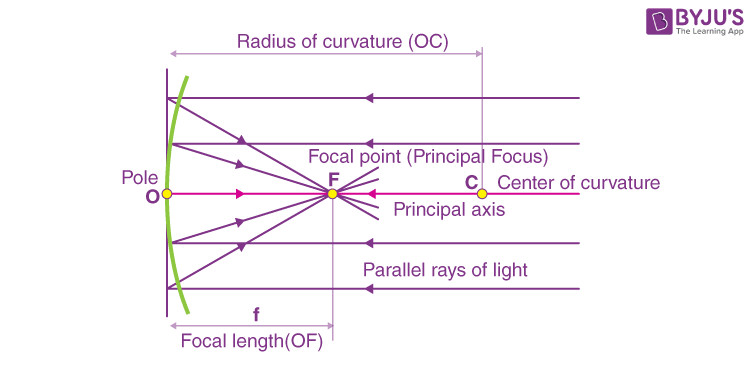## Reflection and Laws of Reflection

Reflection is the phenomenon in which the light travelling in one medium that gets incident on the surface of the second medium returns to the first medium obeying the laws of reflection. The light rays will get reflected from the polished surface.

The laws of reflection are listed below

1. The incident ray, the reflected ray and the normal to the surface at the point of incidence, all lie on the same plane.

2. The angle of incidence will be equal to the angle of reflection.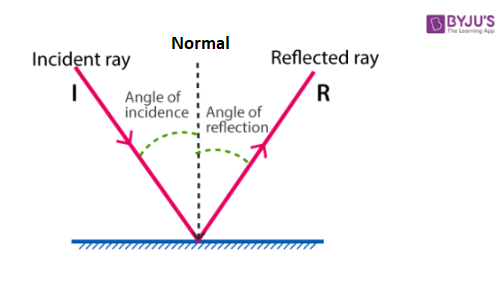## Refraction and Laws of Refraction

Refraction is the phenomenon in which there is a change in the speed of light as it travels from one medium to another, and the consequent bending of a ray of light incident obliquely at the surface of separation of the two media is known as the refraction of light. The following are the laws of refraction.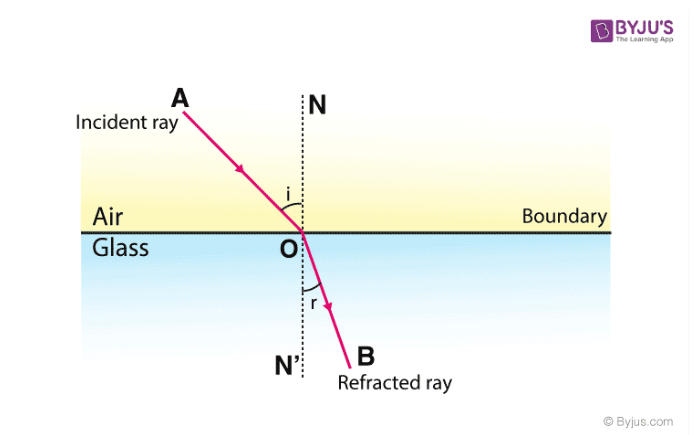1. The incident ray, the refracted ray and the normal to the refracting surface at the point of incidence all lie in the same plane.

2. For a given pair of media and for the light of a given wavelength, the ratio of the sine of the angle of incidence to the sine of the angle of refraction is always a constant.

(sin i/sin r) = constant

This is called Snell’s law. The constant in the equation is called the refractive index of medium 2 with respect to medium 1.

## Factors Affecting Refractive Index

1. The Refractive index of the medium will depend on the nature and temperature of the medium. It also depends on the colour of the light ray.

2. Refractive index is an optical property. Therefore, any impurity added to the medium will alter the refractive index of the medium.

3. The absolute refractive index of the medium is the ratio of the velocity of light in air or vacuum to that in the given medium. The velocity of light is maximum in vacuum. The velocity in any other medium is less than the value in air. Thus, the absolute refractive index of the medium is always greater than unity.

## Reflection from Curved or Spherical Mirror

A spherical mirror is a piece cut from a reflecting sphere. There are two main types of mirrors: convex mirrors and concave mirrors. The reflecting surface of the spherical mirror will be curved inwards or outwards. When the reflecting surface of the spherical mirror is curved inwards it is called a concave mirror, and when it is curved outwards it is called a convex mirror.

### Images Formed by a Concave Mirror

The below diagram shows the ray diagram formed when the object is placed at different positions. In the ray diagrams, let AB be the height of the object and A’B’ be the height of the image for different positions of the object.

1. When the object is beyond the centre of curvature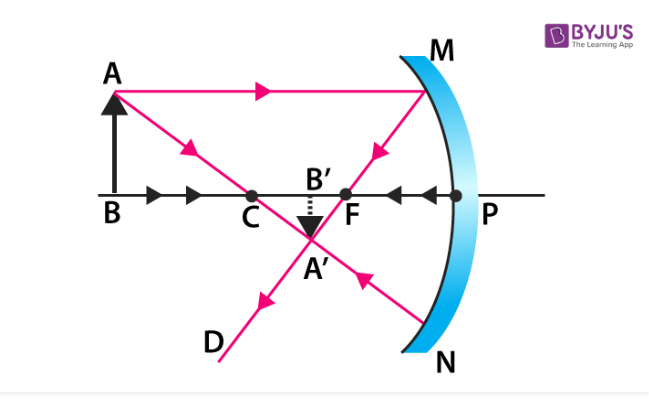2. When the object is at the centre of curvature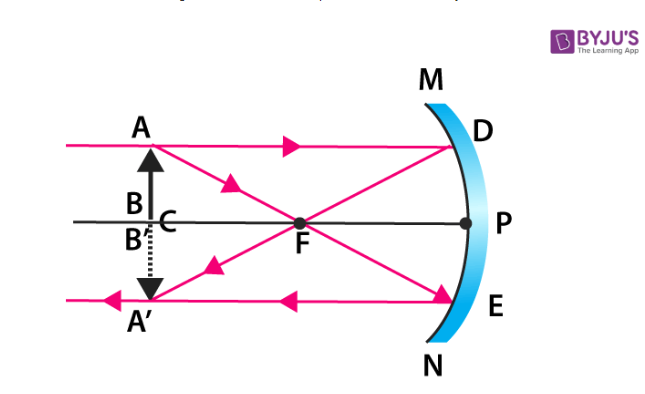3. When the object is at the focus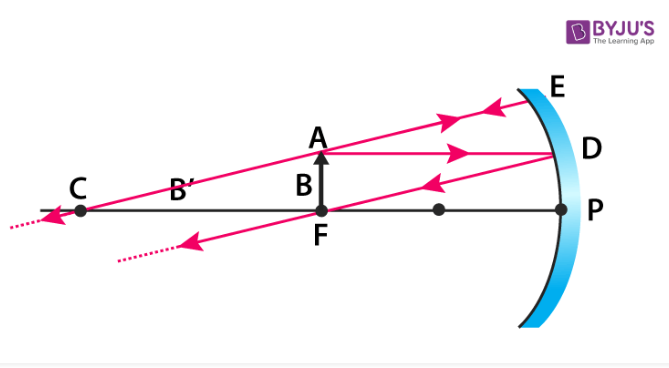4. When the object is at infinity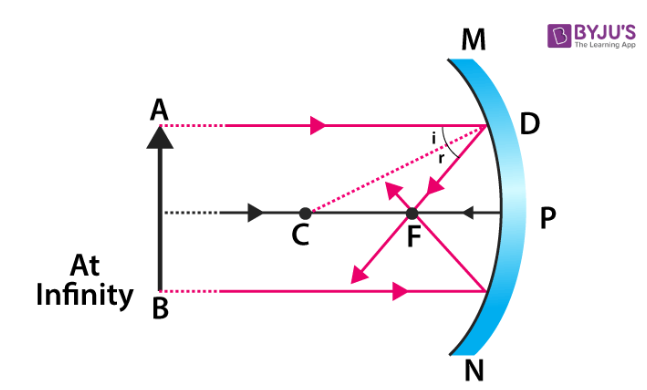5. When the object is between the centre of curvature and the principal focus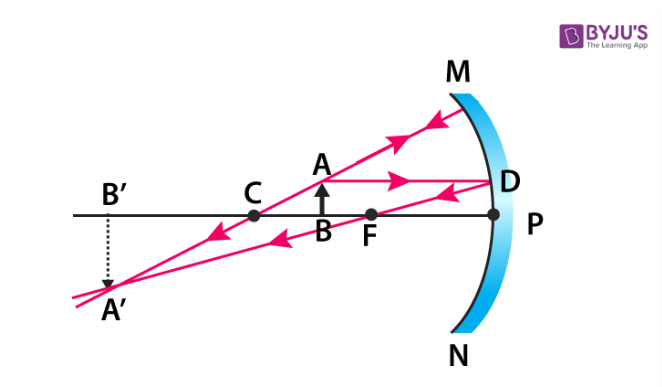6. When the object is between the principal focus and the pole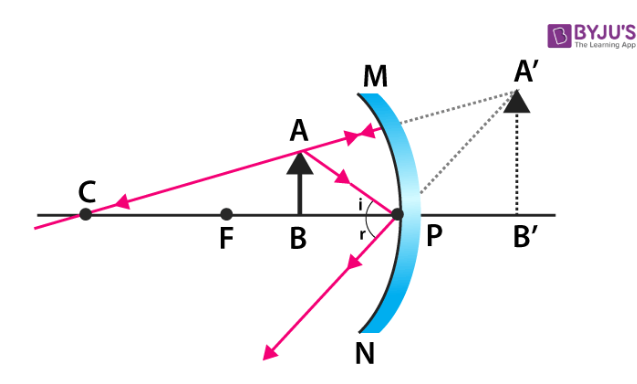### Concave Mirror Object and Image Positions

 Object Position Image Position Nature of Image At Infinity At the principal focus Real, inverted and extremely diminished Beyond the centre of curvature Between the centre of curvature and focus Real, inverted and diminished At the centre of curvature At the centre of curvature Real, inverted, object and image of the same size Between focus and centre of curvature Beyond the centre of curvature Real, inverted, enlarged image At the principal focus At Infinity Extremely magnified Between the pole and principal axis Behind the mirror Virtual, erect and magnified

### Image Formed by a Convex Mirror

The following diagram shows the position and the nature of the image formed by the object placed at different positions.

1. When the object is at infinity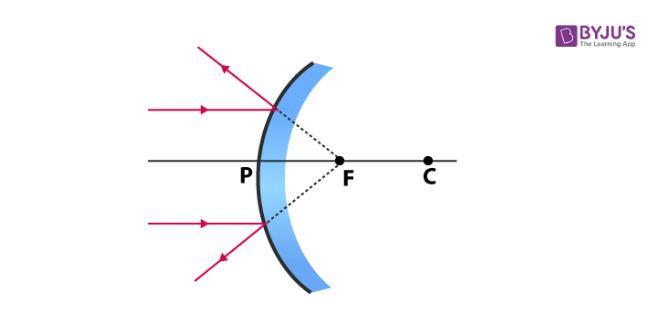1. When the object is at a finite distance from the mirror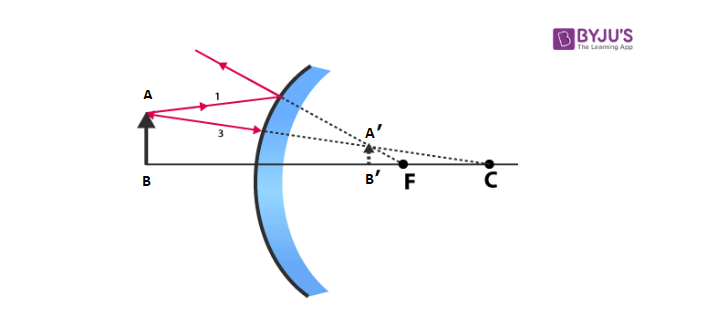### Convex Mirror Object and Image Positions

 Object Position Image Position Nature of Image At Infinity At the principal focus Virtual, erect and extremely diminished Between infinity and pole Appear between focus and pole Virtual, erect and diminished

## Mirror Formula and Magnification

The ratio of the height of the object to the height of the image is called linear magnification.

m = height of the object/height of the image

Linear magnification can also be defined as the ratio of the image distance to the object distance.

If |m| ＜1, it shows that the size of the image is smaller than the object. The negative value of linear magnification shows that the image is real and inverted.

If |m| ＞1, the size of the image is greater than the object.

## Refraction in a Lens

The lens is the most widely used optical device. The lens has two refracting surfaces, if the distance between the two surfaces is small then it is called a thin lens. The lens is of two types: Convex lens and Concave lens. The convex lens is also called the converging lens, and the concave lens is called the diverging lens. The convex lens is thicker at the centre and thinner at the edges. The concave lens is thinner at the centre and thicker at the edges.

## Important Terms in a Lens

1. Optical centre (C): The optical centre is the centre of the lens. The ray of light passing through the optical centre will not get deviated.

2. Principal axis: The line that passes through the centre of curvature and the optical centre is called the principal axis.

3. Centre of curvature: The centre of the sphere of which the lens is a part of is called the centre of curvature.

4. Focal point or focus: The point on the principal axis where all the light rays will meet is known as the focal point.

5. Focal length: The distance between the focus and the pole is called the focal length. The focal length is half of the radius of curvature.

## Image Formed by Convex Lens

The ray diagram for a convex lens is given below

1. When the object is between F and 2F the image is formed beyond 2F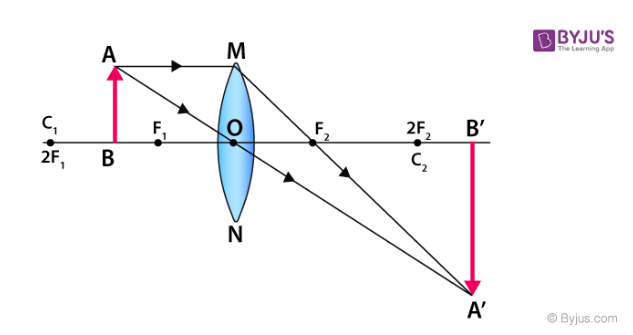2. When the object is at F the image is formed at infinity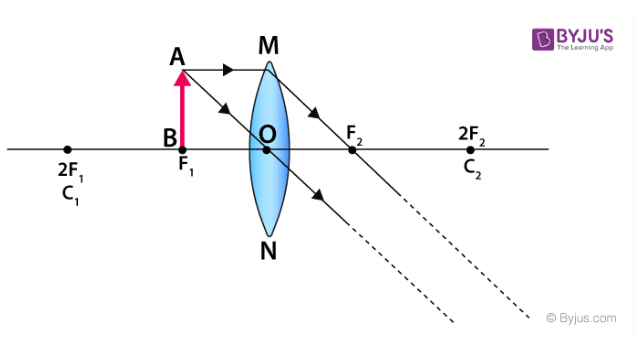3. When the object is placed between focus and the pole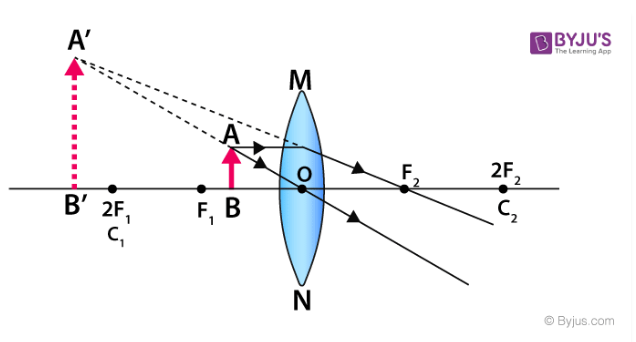4. When the object is at 2F image is also formed at 2F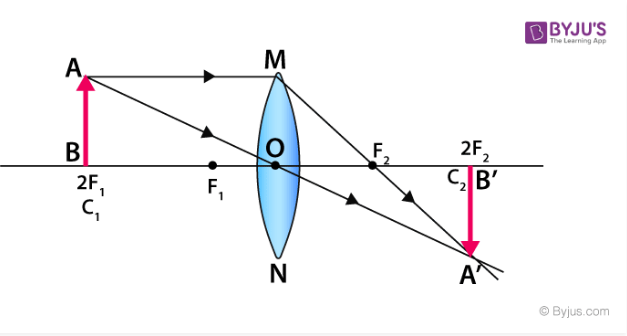5. When an object is behind the 2F the real image is formed between F and 2F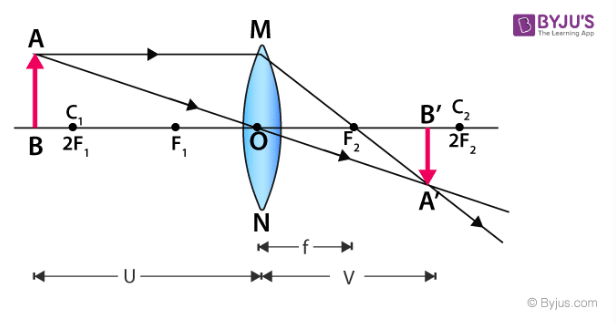6. When the object is at infinity the image is formed at the focus F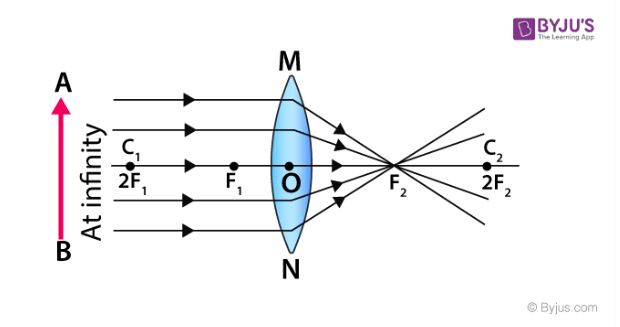Object Position Image Position Nature of Image At Infinity At the focal point Real, inverted and extremely diminished Beyond 2F Between F and 2F Real, inverted and diminished At 2F At 2F Real, inverted, size of image and object is same Between F and 2F Beyond 2F Real, inverted and bigger than object At the principal focus At infinity Real, inverted and extremely magnified Between the optical centre and principal focus Same side as the object Virtual, erect and magnified

## Image Formed by Concave Lens

The nature of the image formed as the object is moved along the principal axis is given below.

1. When the object is at infinity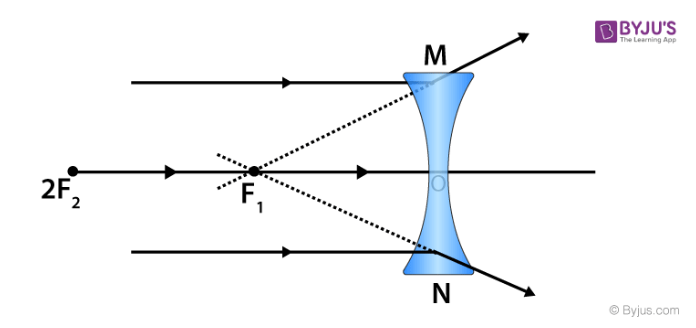1. When the object is between infinity and optical centre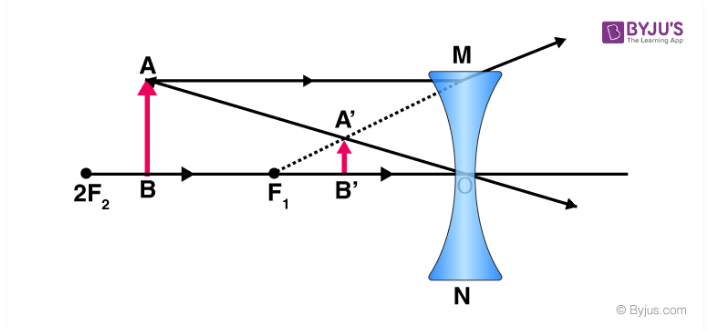Object Position Image Position Nature of Image At Infinity At the principal focus on the same side as the object Virtual, erect and extremely diminished Between infinity and pole Appears between focus and pole Virtual, erect and diminished

## Lens Maker Formula

The lens maker’s formula is the relation between the focal length, the radius of curvature of the lens and the refractive index of the material of the lens. The formula is used by the manufacturers to make the lens of a particular power from the glass of a given refractive index.

The lens maker’s formula is given as

(1/f) = (μ – 1) [(1/R1) – (1/R2)]

f = focal length of the lens

μ = Refractive index

R1 and R2 = radius of curvature of the two surfaces

## Thin Lens Formula

If the thickness of a lens is negligible in comparison to the radius of curvature, it is a thin lens. The following typical equation is used to determine a relation between the focal length of the lens, the distance of the object, and the distance of the image.

(1/f) = (1/v) + (1/u)

f = focal length of the lens

v = distance of the image from the optical centre

u = distance of the object from the optical centre

## Power of Lens

The power of the lens is given by the inverse of focal length.

P = 1/f

The SI unit of the power of the lens is m-1 or diopter.

## Solved Examples

1) An object of 4 cm height is placed at 6 cm in front of a concave mirror of radius of curvature 24 cm. Find the position, height, magnification and nature of the image.

Solution:

h = 4 cm ; u = -6 cm, R = 24 cm, f = ?

v =? h = ?, m = ?

Solution:

Focal length f = R/2 = 24/2 = 12 cm

The mirror equation, 1/f = 1/v + 1/u

1/ -12 = 1/v + 1/-6

1/v = 1/6 – 1/12 = 1/12

Therefore, v = 12 cm

Magnification (m) = h’/h = – v/u

h’ = h ( -v /u ) = 4 ( -12/-6)

Height h’ = 8 cm

m = h’ / h = 8/4 = 2

Magnification m = 2

Image is erect, virtual, twice the height of the object on the right side of the mirror.

2) An object is placed at a certain distance from a convex lens of focal length 20 cm. Find the distance of the object if the image obtained is magnified 4 times.

Solution:

f = 20 cm, m = 4, u = ?

m = v/u = 4

v = 4u

The lens equation is

1/f = 1/v − 1/u

1/f = 1/4u − 1/u

1/f = -3/4u

u = -3f / 4 = − 3 x 20 / 4

u = − 15 cm

## Related Videos

#### Total Internal Reflection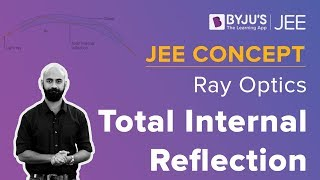#### Important JEE Questions from Ray Optics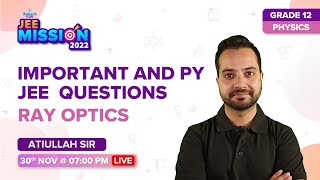#### Most Important Topics for JEE from Ray Optics#### Prism#### Refraction through Lens and Lens Makers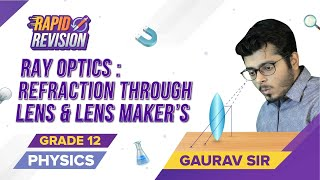#### Combination of Lens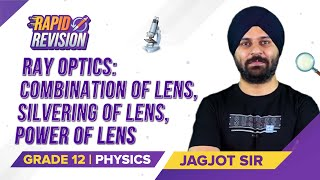#### Refraction Through Spherical Surface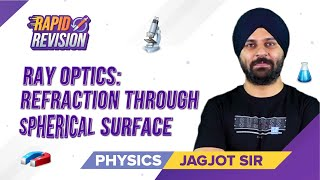#### Lens Makers Formula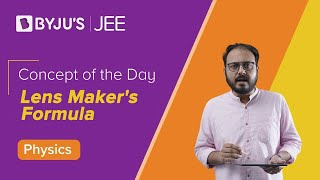#### Ray Optics and Optical Instruments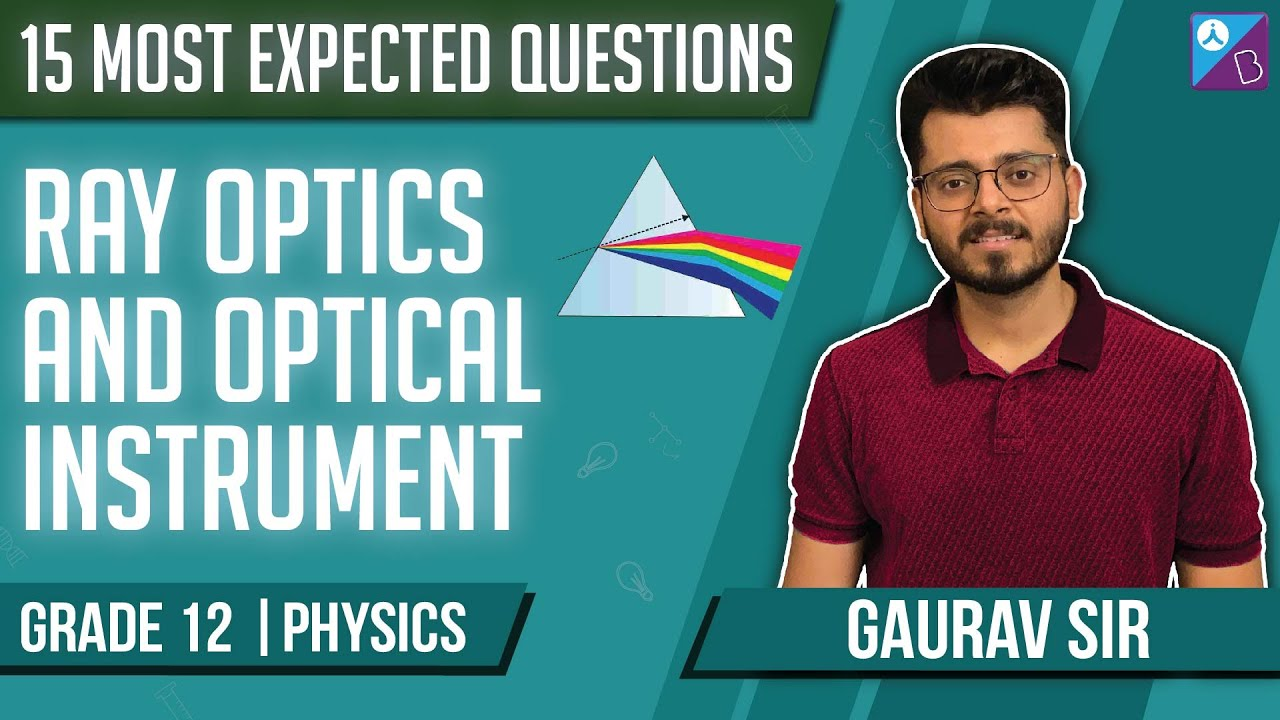## Frequently Asked Questions on Ray optics

### What is Ray optics?

Ray optics also called the geometrical optics is the study of the geometry of the light rays.

### What is the difference between refraction and reflection?

Refraction is the process in which there is a change in the direction of the light rays as it travels from one medium to another, whereas reflection is the change in the direction of the light rays as the ray of light hits another medium and bounces back to the same medium.

### What is the difference between a convex mirror and a concave mirror?

The reflecting surface of the convex mirror curves outwards and the reflecting surface of the concave mirrors curves inwards.

### What is the difference between a lens and a mirror?

Mirrors have one surface that reflects the light. Whereas, the lens has two surfaces, out of which one or both refracts the light.